International
Tables for
Crystallography
Volume B
Reciprocal space
Edited by U. Shmueli

International Tables for Crystallography (2010). Vol. B, ch. 2.5, p. 375   | 1 | 2 |

## Section 2.5.6.7. 3D reconstruction of symmetric objects

B. K. Vainshteinc and P. A. Penczekg

#### 2.5.6.7. 3D reconstruction of symmetric objects

| top | pdf |

Many objects imaged by EM have symmetries; the two types that are often met are helical (phage tails, F-actin, microtubules, myosin thick filaments, and bacterial pili and flagella) and point-group symmetries (many macromolecular assemblies, virus capsids). If the object has helical symmetry, it is convenient to use cylindrical coordinates and a dedicated reconstruction algorithm; particularly for the initial analysis of the data that has to be done in Fourier space. Diffraction from such structures with c period­icity and scattering density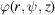is defined by the Fourier–Bessel transform: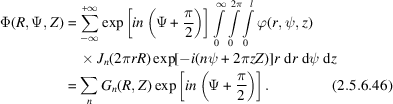The inverse transform has the formso thatandare the mutual Bessel transforms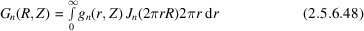and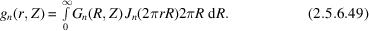Owing to helical symmetry, (2.5.6.48)and (2.5.6.49)contain only those of the Bessel functions that satisfy the selection rule (Cochran et al., 1952)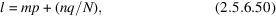where N, q and p are the helix symmetry parameters,. Each layer l is practically determined by the single functionwith the lowest n; the contributions of other functions are neglected. Thus, the Fourier transform of one projection of a helical structure, with an account of symmetry and phases, gives the 3D transform (2.5.6.49). However, biological specimens tend to be flexible and disordered, and exact helical symmetry is rarely observed. A possible approach to dealing with flexibility is to computationally straighten filaments (Egelman, 1986), but this has the potential for introducing artifacts. Another difficulty with helical analysis is that the indexing of a pattern can be ambiguous and the wrong symmetry can be chosen (Egelman & Stasiak, 1988). Further complications exist when the filament does not have a precisely defined helical symmetry, such as F-actin, which has a random variable twist (Egelman et al., 1982). To address these problems, Egelman developed a real-space refinement method for the reconstruction of helical filaments that is capable of determining the helical symmetry of an unknown structure (Egelman, 2000). In this approach, the Fourier–Bessel reconstruction algorithm is replaced by a general reconstruction algorithm discussed in Section 2.5.6.6with the real-space projections supplied as input. Thus, the symmetry is not enforced within the reconstruction algorithm; instead, it is determined and imposed subsequently by real-space averaging.

The presence of point-group symmetry in the structure means that any projection yields as many symmetry-related (but differently oriented) copies of itself as the number of symmetry operations in the group. For example, for three dimensions, each projection enters the reconstruction process at six different projection directions, while for icosahedral symmetry (I) the number is sixty! This high multiplicity makes it possible to write a reconstruction program that explicitly takes into account the symmetry and can perform the task much faster than generic algorithms. Such programs are usually part of dedicated software packages that were specifically designed for the determination of icosahedral structures (Fuller et al., 1996; Lawton & Prasad, 1996; Liang et al., 2002). These icosahedral reconstruction programs are heavily indebted to a set of FORTRAN routines written by R. A. Crowther at the Medical Research Council Laboratory of Molecular Biology (MRC LMB) and perform the 3D reconstruction using the Fourier–Bessel transform strategy outlined above [(2.5.6.46)–(2.5.6.49)] (Crowther, Amos et al., 1970; Crowther & Amos, 1972).

The general reconstruction methods (algebraic, filtered backprojection, direct Fourier inversion) easily accommodate symmetries in the data. In major single-particle reconstruction packages, reconstruction programs are implemented such that the point-group symmetry is a parameter of the program. The symmetry operation is internally taken into account during calculation of the weighting function and it is also applied to the set of Eulerian angles assigned to each projection so the multiple copies are implicitly created and processed. This approach results in an extended time of calculations, but it is entirely general. In direct Fourier inversion algorithms the numerical inaccuracy of the symmetrization performed in Fourier space will result in nonsymmetric artifacts in real space. Thus, in SPIDER (Frank et al., 1996) an additional real-space symmetrization is performed after the reconstruction is completed. Finally, it has to be noted that although it might be tempting to calculate a 3D reconstruction without enforcement of symmetry and to symmetrize the resulting structure, this approach is incorrect. This fact can be seen from the way weighting functions are constructed: weighting functions calculated with symmetries taken into account are not equal to the weighting function calculated for a unique set of projections and subsequently symmetrized.

### References

Cochran, W., Crick, F. H. C. & Vand, V. (1952). The structure of synthetic polypeptides. 1. The transform of atoms on a helix. Acta Cryst. 5, 581–586.
Crowther, R. A. & Amos, L. A. (1972). Three-dimensional image reconstructions of some small spherical viruses. Cold Spring Harbor Symp. Quant. Biol. 36, 489–494.
Crowther, R. A., Amos, L. A., Finch, J. T., DeRosier, D. J. & Klug, A. (1970). Three dimensional reconstruction of spherical viruses by Fourier synthesis from electron micrographs. Nature (London), 226, 421–425.
Egelman, E. (1986). An algorithm for straightening images of curved filamentous structures. Ultramicroscopy, 19, 367–374.
Egelman, E. H. (2000). A robust algorithm for the reconstruction of helical filaments using single-particle methods. Ultramicroscopy, 85, 225–234.
Egelman, E. H., Francis, N. & DeRosier, D. J. (1982). F-actin is a helix with a random variable twist. Nature (London), 298, 131–135.
Egelman, E. H. & Stasiak, A. (1988). Structure of helical RecA–DNA complexes. II. Local conformational changes visualized in bundles of RecA–ATP gamma S filaments. J. Mol. Biol. 200, 329–349.
Frank, J., Radermacher, M., Penczek, P., Zhu, J., Li, Y., Ladjadj, M. & Leith, A. (1996). SPIDER and WEB: processing and visualization of images in 3D electron microscopy and related fields. J. Struct. Biol. 116, 190–199.
Fuller, S. D., Butcher, S. J., Cheng, R. H. & Baker, T. S. (1996). Three-dimensional reconstruction of icosahedral particles – the uncommon line. J. Struct. Biol. 116, 48–55.
Lawton, J. A. & Prasad, B. V. V. (1996). Automated software package for icosahedral virus reconstruction. J. Struct. Biol. 116, 209–215.
Liang, Y. Y., Ke, E. Y. & Zhou, Z. H. (2002). IMIRS: a high-resolution 3D reconstruction package integrated with a relational image database. J. Struct. Biol. 137, 292–304.